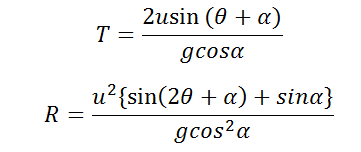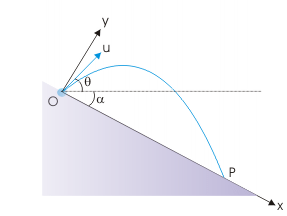# Parameters of a Projectile on an incline moving downwards Calculator

This CalcTown calculator calculates the parameters (i.e time of flight and range) of a Projectile projected downwards on an incline.

m/s

#### Result

s
mClick here to view image

where

θ=Angle of Projection of the body w.r.t horizontal

α=Angle of the incline

u=Projection velocity